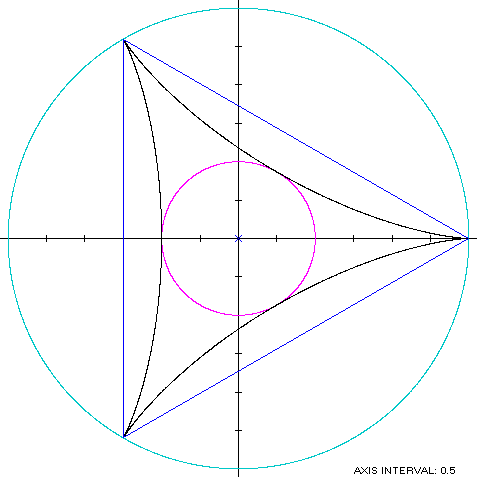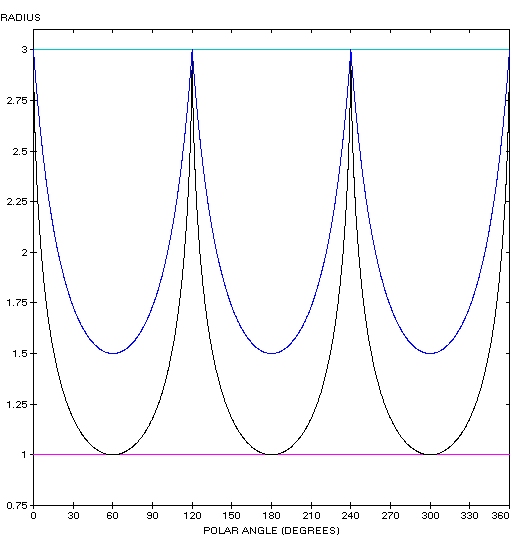Deltoid
DELTOID

Balmoral Software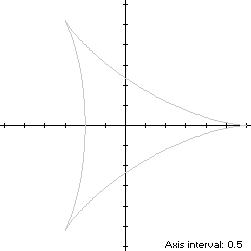The deltoid is a three-cusped hypocycloid S with parametric equations
x(t) = 2cos(t) + cos(2t)

y(t) = 2sin(t) - sin(2t), 0 ≤ t < 2π

The curve is traversed in a counterclockwise direction, starting at the right cusp (3,0) at t = 0, reaching the next cusp at t = 2π/3 and then continuing symmetrically around the origin. Since S has two minimum-abscissa points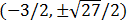, it is non-convex by the multiple local extrema test. The width x height of its bounding rectangle is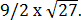### Symmetry

Since
x(t + 2π/3) = cos(2π/3)x(t) - sin(2π/3)y(t)
and
y(t + 2π/3) = sin(2π/3)x(t) + cos(2π/3)y(t),
the conditions (R1) of Lemma R are satisfied and the deltoid is rotationally symmetric with period 2π/3.

### Metrics

The perimeter of the deltoid is 16 and its area is 2π.

### Boundary Circles & Circumellipse

Since the deltoid is rotationally symmetric with period 2π/3, its circumcircle and circumellipse are the same. The squared-distance function of S
x2(t) + y2(t) = 4cos(3t) + 5
ranges from 1 to 9, so its inradius is 1 and its circumradius is 3.

### Inellipse

Using z = -1 in Lemma E,
d/dt [x(t) - z]y(t) = d/dt 4sin3(t)cos(t)
has a zero at t = ±π/3. The corresponding points on S are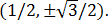Together with (z,0) = (-1,0), these points are all at a unit distance from the origin, so an axis-aligned ellipse tangent to S at (-1,0) is a unit circle by Lemma S. Therefore, the inellipse is the same as the incircle.

### Convex Hull

The convex hull is an equilateral triangle created by connecting the maximum-radius points (3,0) andby line segments of length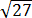, as shown in blue in the left diagram below. The perimeter of the convex hull is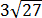, which is about 3% shorter than that of the deltoid. The area of the convex hull is half that of its bounding rectangle: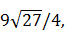and is about 86% more than the area of the deltoid.

### Summary Table

 Perimeter Area Centroid Figure Parameters Incircle R = 1 6.283185 3.141593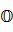Deltoid16 6.283185 Convex hull 15.588457 11.691343 Circumcircle R = 3 18.849556 28.274334